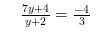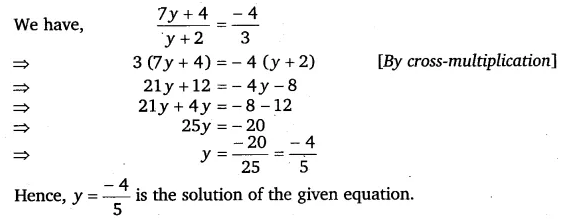# Solve the following equations: 7y + 4/y+2 = -4/3

Solve the following equations: